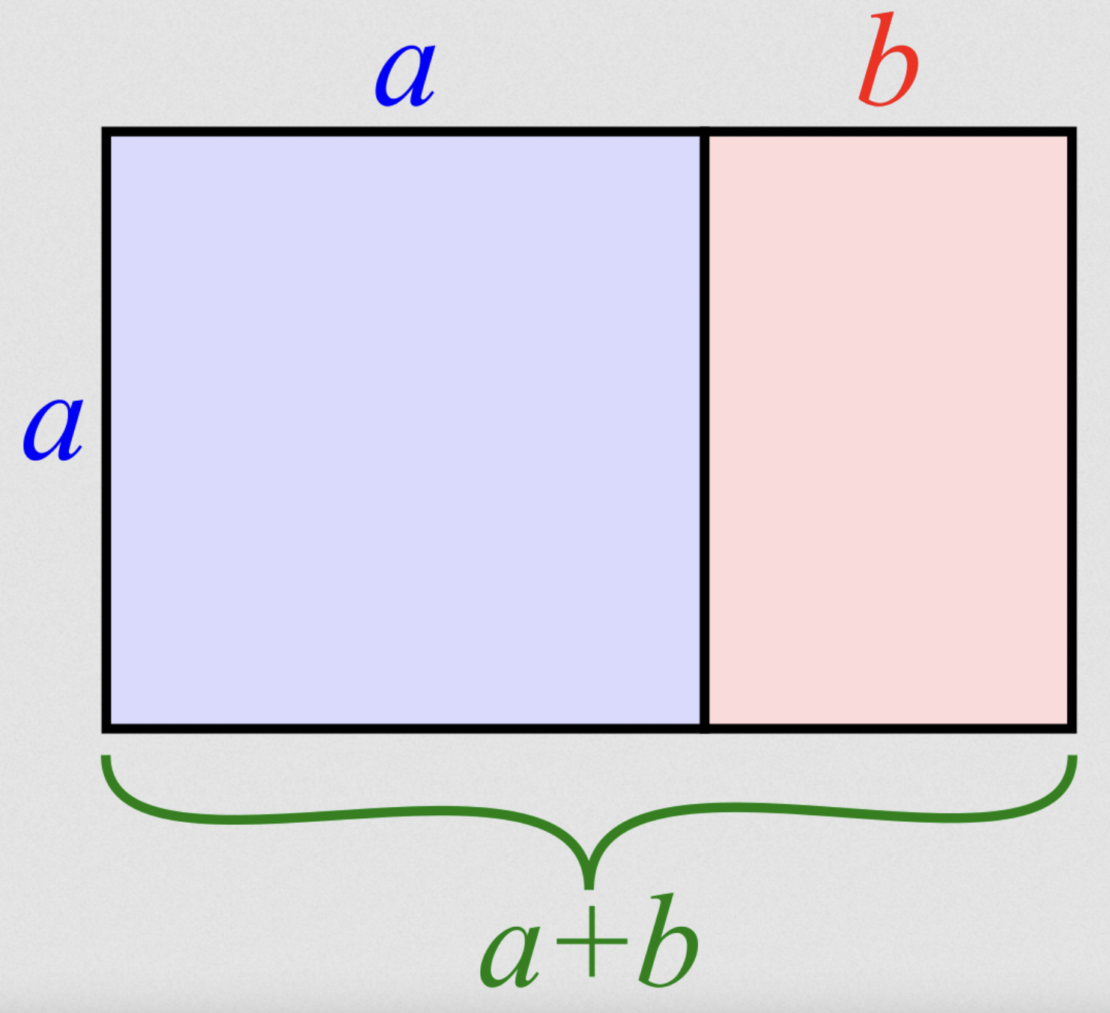# What Fibonacci, and Ancient Indian Scholars, Didn’t Know About the Golden Ratio

•Many indocentric claims about the Fibonacci series and the ‘golden ratio’ in mathematics don’t do justice to the actual history of the subject. •It is easy these days to find articles and social media posts claiming to analyse the relationship between the Fibonacci series and golden ratio, and thereon to Indian culture. •More often than not, such claims are used to float deceptive conclusions about how ancient Indian scholars knew these concepts and their applications.

Many indocentric claims about the Fibonacci series and the ‘golden ratio’ in mathematics don’t do justice to the actual history of the subject – while the proponents of these claims almost always exaggerate their assertions to a point where many original innovators don’t get their due credit.

The Fibonacci series is one of many interesting topics in mathematics, most importantly for its association with the golden ratio, which has a lot of peculiar properties and an equal number of myths related to it. You can obtain the Fibonacci series by taking two initial numbers, say 0 and 1, and then calculating the next term by adding the two previous terms. So the series goes thus:

0, 1, 1, 2, 3, 5, 8, 13, 21, …

The Fibonacci series also occurs throughout nature, like in the arrangement of certain seed patterns.

Through this article, let’s examine some historical arguments associated with the Fibonacci series, the golden ratio and their relationship – and to claims about work on these concepts by scholars in ancient India.

A line is said to be divided in the golden ratio if the ratio of the larger to the smaller part is as the whole to the larger part. As simple as it may sound, the implications of such a definition are profound. One is the self-similarity property of the golden ratio. This is obvious if you take the ‘golden rectangle’ (image below) and divide it according to the golden ratio. The part of the rectangle that remains will itself have sides whose lengths are proportionate to the golden ratio.###### And if you continued this process of division as above, you will obtain a series of ever smaller rectangles whose sides are according to the golden ratio itself.

As the 17th century German astronomer Johannes Kepler wrote:

A peculiarity of this proportion lies in the fact that a similar proportion can be constructed out of the larger part and the whole; what was formerly the larger part now becomes the smaller, what was formerly the whole now becomes the larger part, and the sum of these two now has the ratio of the whole. This goes on indefinitely; the divine proportion always remaining…

The next interesting point is the relationship between the Fibonacci series and the golden ratio. Specifically, the ratio of consecutive terms of the series converges to the golden ratio as the number of terms are increased. For a substantial part of the history of the Fibonacci series, mathematicians didn’t recognise this relationship.

It is easy these days to find articles and social media posts claiming to analyse the relationship between the Fibonacci series and golden ratio, and thereon to Indian culture. More often than not, such claims are used to float deceptive conclusions about how ancient Indian scholars knew these concepts and their applications – at the same time without ever discussing these scholars’ work.

Most of these claims spiral out from one established fact that Sanskrit scholars, led by Pingala (c. second century BC), investigated a series that later came to be called the ‘Fibonacci series’, with reference to Sanskrit prosody. The claimants here typically make four mistakes:

1. The Fibonacci series and the golden ratio are not the same thing, and for centuries mathematicians didn’t know about how they were related.

2. Some have said Fibonacci (a.k.a. Leonardo of Pisa) credited ancient Hindu scholars for the series named after him. However, Fibonacci appreciated the Hindu algorithms in his famous work on computations, Liber Abaci (1202), but didn’t credit the series to anyone. His approach also seems original and significantly different from the one Pingala employed.

3. Fibonacci himself didn’t know about the relationship between the series named after him and the golden ratio, although he worked with both of them. This relationship came to light in 1560, in the work of the German mathematician Simon Jacob, and followed by Kepler’s independent discovery in 1608.

4. Hindu scholars didn’t record the appearance of the golden ratio and the Fibonacci series in nature. These breakthroughs came later in medieval and modern Europe.